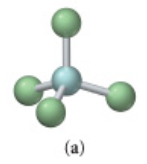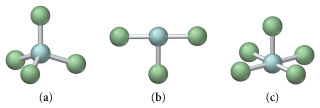# Problem: The following figures show several molecular geometries.Give the number of total electron groups, the number of bonding groups, and the number of lone pairs for (a) geometry.

###### FREE Expert Solution
• Analyzing structure A, A appears to have 4 groups attached on the central atom.
• So this will have  4 electron groups which are all bonding groups
• This structure is  tetrahedral which is  AX4 (A is central atom, X is the substituents)• B appears to be a T-shaped molecule with 3 groups on it but AXdoesnt follow a T-shape geometry. AX3 follows a trigonal planar geometry.
92% (312 ratings)###### Problem Details

The following figures show several molecular geometries.Give the number of total electron groups, the number of bonding groups, and the number of lone pairs for (a) geometry.